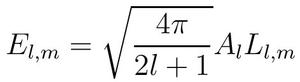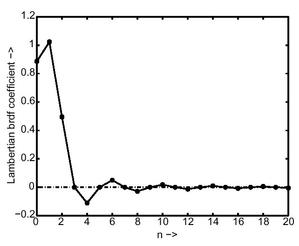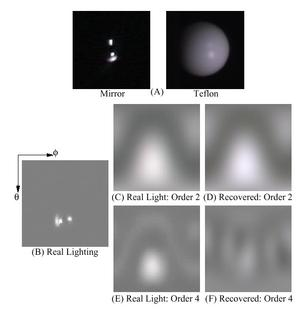# On the Relationship between Radiance and Irradiance: Determining the illumination from images of a convex Lambertian object

### Abstract

A practical realization of the radiance-from-irradiance problem is the estimation of the lighting from images of a homogeneous convex curved Lambertian surface of known geometry under distant illumination, since a Lambertian object reflects light equally in all directions proportional to the irradiance. We briefly discuss practical and physical considerations, and describe a simple experimental test to verify our theoretical results.

### Summary

This paper derives an analytic formula for the irradiance in terms of the radiance. The formula is in terms of spherical harmonics which can be thought of in some sense as the eigenfunctions of the Lambertian BRDF. It is shown that the odd-order modes of the illumination (greater than one) are completely annihilated, so irradiance cannot be estimated from radiance. There is also a deeper analysis of how positivity of the lighting affects the results, and how this can allow for a solution in certain special cases.

Finally, we present empirical and theoretical evidence showing that in practice, the irradiance depends only on the first 2 orders of spherical harmonic modes of the illumination, and can be represented as a quadratic polynomial of the cartesian components of the surface normal. In fact, 99% of the energy of the Lambertian BRDF filter is contained by orders 0,1, and 2. In particular, we care only about the first 2 orders of the illumination, that is 9 parameters. These first 9 coefficients are also all that can be determined regarding the lighting. This 9 parameter model is likely to have wide impact in computer graphics and vision, as we have already demonstrated for forward and inverse rendering.

### Results

The figures on the right summarize some of the main results from the paper.

Figures 1,2: Our analytic irradiance formula in terms of spherical harmonic coefficients, and the analytic values for the coefficients of the Lambertian BRDF filter. These are the key results of the paper. The coefficients of the Lambertian BRDF fall off as l^(-5/2) for even terms and vanish for odd terms > 1. This makes radiance-from-irradiance formally ill-posed as well as ill-conditioned for frequencies l > 2.

Figure 3: A plot of the spherical harmonic coefficients (from the equations in figure 2) versus frequency (l). We see the rapid decay of coefficients and the zeros for odd modes greater than one.

Figure 4: Showing that we can indeed get the first 2 orders (9 terms) of the lighting from the irradiance but that this fails miserably for higher orders.

• JOSA 2001 paper in PDF (.4M)
Correction: In equation 19, there is a small misprint. The last term should be ((n/2)!)^2, not (n!/2)^2
• Related Siggraph paper on environment mapping of diffuse objects using the 9 parameter approximation.Figure 1. The analytic formula for irradiance in terms of spherical harmonic coefficients.Figure 2. Analytic formulae for spherical harmonic coefficients of Lambertian BRDF filter.Figure 3. Plot of spherical harmonic terms in Lambertian BRDF filter.Figure 4. Comparing recovered illumination for orders 2 and 4 from observations of the irradiance (a near-Lambertian object) to actual lighting.

Ravi Ramamoorthi